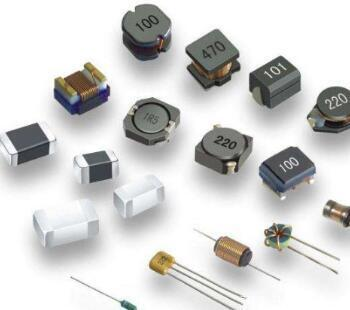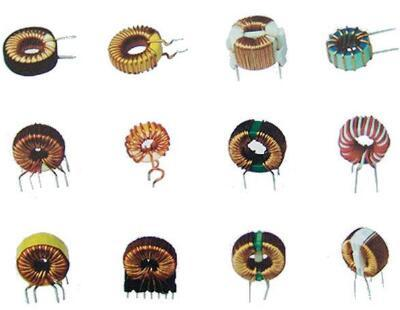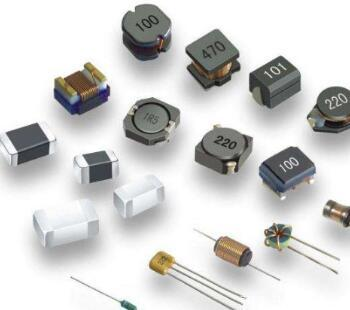# Magnetic Inductors

##### author: MagTop
02/04/2022# 1.  Define

The Inductor is a device that converts electric energy into magnetic energy and stores it based on the principle of electromagnetic induction. In fact, all devices that can produce self-inductance and mutual inductance can be called inductors. Inductor has a wide range of uses, in the AC Circuit inductor has the ability to block the AC through, in the circuit is often used as resistance, voltage, AC coupling and load; When the inductor and capacitor are combined, they can be used for tuning, filtering, frequency selection, frequency division,etc. The simplest inductor is one with a hollow conductor wound several times. Inductors with a magnetic core coil several times with a conductor wound over the core. No matter which kind of inductor, if the structure is the same, its basic characteristics are the same, but the number of turns is different or the core is different, the inductance of the inductor is different. The more turns the coil has, the greater the inductance will be. In the same number of turns, the inductance will increase after the core is added to the coil. Inductors have inductive reactance properties in a circuit, which act as resistance to the flow of current, but which are related to the frequency of the current flowing through the inductor, as well as to the amount of inductance of the inductor itself. Inductance is a path for DC, but a great obstacle for AC, which is usually called inductive reactance, XL=2πfL, where XL is the inductive reactance of inductance; F is the frequency of the current flowing through the inductive ac; L is the amount of inductance of inductance. On the premise that the inductance is determined, the greater the inductance is, the greater the hindrance is.2. Working principle
When an electric current passes through a wire, it creates a concentric magnetic field centered around the wire. At this point, if the wire is bent into the "spring shape" shown in the figure, themagnetic flux inside the inductor will point in the same direction, thus increasing. By adjusting the number of turns, a magnetic field proportional to the number of turns can be generated. That's how the inductor works. A current passing through an inductor creates a magnetic field, whereas a change in the magnetic field creates a current. (Law of electromagnetic induction)
E = L (d i/d t)
L: Self-induction of inductor
E: Counter electromotive force

2-1> Principle of inductor
The counter electromotive force (E) generated in the inductor is proportional to the rate of change of current per unit time (DI /dt) and therefore does not occur when a direct current continues to flow in the same direction. In other words, the inductor has no effect on the DC current, only the ac current blocking function. Using this property of the inductor, it can be used as a resistance (impedance) in ac circuits. The impedance Z(unit ω) of the inductor is:
Z = L = 2 PI omega fL
F is the ac frequency and L is the inductor self-induction.

3.
Symbols and units
(1) Inductor symbol: L
(2) Inductance unit: H, mH, uH, 1H=103mH=106uH.
(3) nominal inductance: direct standard, color ring standard and nostandard
(4) Inductance directivity: no direction
(5) Check the quality of inductance method: use inductance measuring instrument to measure the inductance; When the multimeter is used to measure its on-off, the ideal inductance resistance is very small, almost zero.

4. Main technical parameters
(1) Inductance: also known as self-inductance coefficient (L), is a physical quantity that represents the self-inductance capacity of inductance components. The unit of L is H(H), mH(millih) and μH (micro H). The conversion relation of the three is as follows: 1H = 103mH = 106μH

(2)Quality factor: is the inductance coil quality parameters, also known as Q value or optimal value. The higher the Q value, the lower the loss of the circuit, the higher the efficiency.

(3)Distributed capacitance: coil turns, between the coil and the
ground, between the coil and the shielding box and between the layers of the coil capacitance, collectively known as the distributed capacitance of the coil. The existence of distributed capacitance will increase the equivalent total loss resistance and decrease the quality factor Q.

(4) Rated current: refers to the maximum working current allowed through the coil for a long time.

(5) Stability: mainly refers to the extent to which parameters are affected by temperature, humidity and mechanical vibration.